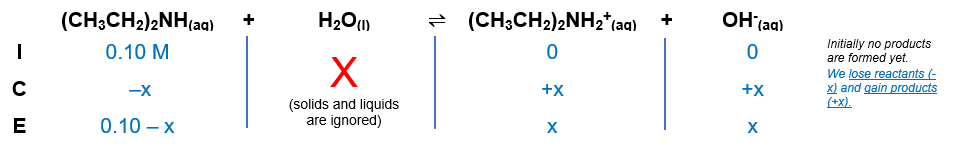# Problem: What is the pH of 0.10M diethylamine, (CH3CH2)2NH, (Kb=8.6x10-4)?

###### FREE Expert Solution

Equilibrium reaction:        (CH3CH2)2NH(aq) + H2O(l)  (CH3CH2)2NH2+(aq) + OH-(aq)

Step 1: Construct an ICE chart for the reaction.Step 2: Write the Kb expression and calculate for Kb.

$\overline{){{\mathbf{K}}}_{{\mathbf{b}}}{\mathbf{=}}\frac{\mathbf{products}}{\mathbf{reactants}}}\phantom{\rule{0ex}{0ex}}{\mathbf{K}}_{\mathbf{b}}\mathbf{=}\frac{\left[{\left(\mathrm{CH}}_{}}{}$3CH2)2NH2+][OH-][CH3CH22NH]

Solids and liquids are not included in the expression

Step 3: Calculate the equilibrium concentrations.

${\mathbf{K}}_{\mathbf{b}}\mathbf{=}\frac{\left[\mathbf{x}\right]\left[\mathbf{x}\right]}{\left[\mathbf{0}\mathbf{.}\mathbf{10}\mathbf{-}\mathbf{x}\right]}\phantom{\rule{0ex}{0ex}}\overline{){{\mathbf{K}}}_{{\mathbf{b}}}{\mathbf{=}}\frac{{\mathbf{x}}^{\mathbf{2}}}{\mathbf{0}\mathbf{.}\mathbf{10}\mathbf{-}\mathbf{x}}}$

We cannot remove (–x) from the equation

$\overline{){\mathbf{x}}{\mathbf{=}}\frac{\mathbf{-}\mathbf{b}\mathbf{±}\sqrt{{\mathbf{b}}^{\mathbf{2}}\mathbf{-}\mathbf{4}\mathbf{a}\mathbf{c}}}{\mathbf{2}\mathbf{a}}}$

97% (449 ratings)###### Problem Details

What is the pH of 0.10M diethylamine, (CH3CH2)2NH, (Kb=8.6x10-4)?